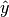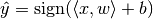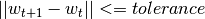# Classification¶

A classification example explained by the usage of SALSA package on the Ripley data set. The SALSA package provides many different options for stochastically learning a classification model.

This package provides a function salsa and explanation on SALSAModel which accompanies and complements it. The package provides full-stack functionality including cross-validation of all model- and algorithm-related hyperparameters.

## Knowledge agnostic usage¶

salsa(X, Y[, Xtest])

Create a linear classification model with the predicted output:based on data given in X and labeling specified in Y. Optionally evaluate it on Xtest. Data should be given in row-wise format (one sample per row). The classification model is embedded into the returned model as model.output. The choice of different algorithms, loss functions and modes will be explained further on this page.

using SALSA, MAT, Base.Test

srand(1234)

model = salsa(ripley["X"], ripley["Y"], ripley["Xt"]) # --> SALSAModel(...)
@test_approx_eq_eps mean(ripley["Yt"] .== model.output.Ytest) 0.89 0.01

salsa(mode, algorithm, loss, X, Y, Xtest)

Create a classification model with the specified choice of algorithm, mode and loss function.

Parameters: mode – LINEAR vs. NONLINEAR mode specifies whether to use a simple linear classification model or to apply the Nyström method for approximating the feature map before proceeding with the learning scheme algorithm – stochastic algorithm to learn a classification model, e.g. PEGASOS, L1RDA etc. loss – loss function to use when learning a classification model, e.g. HINGE, LOGISTIC etc. X – training data (samples) represented by Matrix or SparseMatrixCSC Y – training labels Xtest – test data for out-of-sample evaluation SALSAModel object.
using SALSA, MAT, Base.Test

srand(1234)

model = salsa(LINEAR, PEGASOS, HINGE, ripley["X"], ripley["Y"], ripley["Xt"])
@test_approx_eq_eps mean(ripley["Yt"] .== model.output.Ytest) 0.89 0.01


## Model-based usage¶

salsa(X, Y, model, Xtest)

Create a classification model based on the provided model and input data

Parameters: X – training data (samples) represented by Matrix or SparseMatrixCSC Y – training labels Xtest – test data for out-of-sample evaluation model – model is of type SALSAModel{L <: Loss, A <: Algorithm, M <: Mode, K <: Kernel} and can be summarized as follows (with default values for named parameters):
• mode::Type{M}: mode used to learn the model: LINEAR vs. NONLINEAR (mandatory parameter)
• algorithm::A: algorithm used to learn the model, e.g. PEGASOS (mandatory parameter)
• loss_function::Type{L}: type of a loss function used to learn the model, e.g. HINGE (mandatory parameter)
• kernel::Type{K} = RBFKernel: kernel used in NONLINEAR mode to compute Nyström approximation
• global_opt::GlobalOpt = CSA(): global optimization techniques for tuning hyperparameters
• subset_size::Float64 = 5e-1: subset size used in NONLINEAR mode to compute Nyström approximation
• max_cv_iter::Int = 1000: maximal number of iterations (budget) for any algorithm in training CV
• max_iter::Int = 1000: maximal number of iterations (budget) for any algorithm for final training
• max_cv_k::Int = 1: maximal number of data points used to compute loss derivative in training CV
• max_k::Int = 1: maximal number of data points used to compute loss derivative for final training
• online_pass::Int = 0: if > 0 we are in the online learning setting going through the entire dataset online_pass times
• normalized::Bool = true: normalize data (extracting mean and std) before passing it to CV and final learning
• process_labels::Bool = true: process labels to comply with binary (-1 vs. 1) or multi-class classification encoding
• tolerance::Float64 = 1e-5: the criterion is evaluated for early stopping (online_pass==0)• sparsity_cv::Float64 = 2e-1: sparsity weight in the combined cross-validation/sparsity criterion used for the RDA type of algorithms
• validation_criterion = MISCLASS(): validation criterion used to verify the generalization capabilities of the model in cross-validation
Returns: SALSAModel object with model.output of type OutputModel structured as follows:
• dfunc::Function: loss function derived from the type specified in loss_function::Type{L} (above)
• alg_params::Vector: vector of model- and algorithm-specific hyperparameters obtained via cross-validation
• X_mean::Matrix: row (vector) of extracted column-wise means of input X if normalized::Bool = true
• X_std::Matrix: row (vector) of extracted column-wise standard deviations of input X if normalized::Bool = true
• mode::M: mode used to learn the model: LINEAR vs. NONLINEAR
• w: found solution vector (matrix)
• b: found solution offset (bias)
using SALSA, MAT, Base.Test

srand(1234)